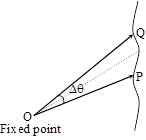# Circular Motion & Rotational Dynamics

#### 1. Angular Displacement

1. Angular displacement
Introduction : Angle subtended by position vector of a particle moving along any arbitrary path w.r.t. some fixed point is called angular displacement.(a) Particle moving in an arbitrary path(b) Particle moving in straight line(c) Particle moving in circular path
(i) Angular displacement is a vector quantity.
(ii) Its direction is perpendicular to plane of rotation and given by right hand screw rule.
Note: Clockwise angular displacement is taken as negative and anticlockwise displacement as positive.(iii) For circular motion ΔS = r × Δq
(iv) Its unit is radian (in M.K.S)
Note : Always change degree into radian, if it occurs in numerical problems.
Note : 1 radian =⇒ π radian = 180°
(v) It is a dimensionless quantity i.e. dimension [M0L0T0]

If you want to give information about online courses to other students, then share it with more and more on Facebook, Twitter, Google Plus. The more the shares will be, the more students will benefit. The share buttons are given below for your convenience.

#### IIT (Class X)

• Unit, Dimension & Error
• Vectors
• Motion in One Dimension
• PROJECTILE MOTION
• NEWTON'S LAWS OF MOTION & FRICTION
• WORK, POWER, ENERGY & CONSERVATION LAWS
• CIRCULAR MOTION & ROTATIONAL DYNAMICS
• GRAVITATION